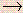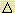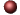KineticsExamples ofMultiple Choice Questions

1.
The combustion of ethane (C2H6) is represented by the equation:
2C2H6(g) + 7O2(g)4CO2(g) + 6H2O(l)
In this reaction:
(a) the rate of consumption of ethane is seven times faster than the rate of consumption of oxygen.
(b) the rate of formation of CO2 equals the rate of formation of water.
(c) water is formed at a rate equal to two-thirds the rate of formation of CO2.
(d) the rate of consumption of oxygen equals the rate of consumption of water.
(e) CO2 is formed twice as fast as ethane is consumed.

2.
The speed of a chemical reaction
(a) is constant no matter what the temperature is.
(b) is independent of the amount of contact surface of a solid involved.
(c) between gases should in all cases be extremely rapid because the average kinetic energy of the molecules is great.
(d) between ions in aqueous solution is extremely rapid because there are no bonds that need to be broken.
(e) varies inversely with the absolute temperature.

3.
For a reaction 2A + B2C, with the rate equation: Rate = k[A]2[B]
(a) the order with respect to A is 1 and the order overall is 1.
(b) the order with respect to A is 2 and the order overall is 2.
(c) the order with respect to A is 2 and the order overall is 3.
(d) the order with respect to B is 2 and the order overall is 2.
(e) the order with respect to B is 2 and the order overall is 3.

4.
Given the following data for this reaction:
NH4+(aq) + NO2-(aq)N2(g) + 2H2O(l)
 EXPT [NH4+] [NO2-] RATE 1 0.010 M 0.020 M 0.020 M/s 2 0.015 M 0.020 M 0.030 M/s 3 0.010 M 0.010 M 0.005 M/s
The rate law for the reaction is:
(a) Rate = k[NH4+][NO2-]
(b) Rate = k[NH4+]2[NO2-]2
(c) Rate = k[NH4+]2[NO2-]
(d) Rate = k[NH4+][NO2-]2
(e) none of the above

5.
What are the units of k for the rate law: Rate = k[A][B]2, when the concentration unit is mol/L?
(a) s-1
(b) s
(c) L mol-1 s-1
(d) L2 mol-2 s-1
(e) L2 s2 mol-2

6.
Given: A + 3B2C + D
This reaction is first order with respect to reactant A and second order with respect to reactant B. If the concentration of A is doubled and the concentration of B is halved, the rate of the reaction would _____ by a factor of _____.
(a) increase, 2
(b) decrease, 2
(c) increase, 4
(d) decrease, 4
(e) not change

7.
The decomposition of carbon disulfide, CS2, to carbon monosulfide, CS, and sulfur is first order with k = 2.8 x 10-7 s-1 at 1000oC.
CS2CS + S
What is the half-life of this reaction at 1000oC?
(a) 5.0 x 107 s
(b) 4.7 x 10-6 s
(c) 3.8 x 105 s
(d) 6.1 x 104 s
(e) 2.5 x 106 s

8.
The decomposition of dimethylether at 504 oC is first order with a half-life of 1570 seconds. What fraction of an initial amount of dimethylether remains after 4710 seconds?
(a) 1/3
(b) 1/6
(c) 1/8
(d) 1/16
(e) 1/32

9.
The half-life for a first-order reaction is 32 s. What was the original concentration if, after 2.0 minutes, the reactant concentration is 0.062 M?
(a) 0.84 M
(b) 0.069 M
(c) 0.091 M
(d) 0.075 M
(e) 0.13 M

10.
Given that a reaction absorbs energy and has an activation energy of 50 kJ/mol, which of the following statements are correct? (Hint: Draw the potential energy diagram.)
(1) The reverse reaction has an activation energy equal to 50 kJ/mol.
(2) The reverse reaction has an activation energy less than 50 kJ/mol.
(3) The reverse reaction has an activation energy greater than 50 kJ/mol.
(4) The change in internal energy is less than zero.
(5) The change in internal energy is greater than zero.

(a) (1) and (4)
(b) (2) and (4)
(c) (3) and (4)
(d) (2) and (5)
(e) (3) and (5)

11.
If reaction A has an activation energy of 250 kJ and reaction B has an activation energy of 100 kJ, which of the following statements must be correct?
(a) If reaction A is exothermic and reaction B is endothermic then reaction A is favored kinetically.
(b) At the same temperature the rate of reaction B is greater than the rate of reaction A.
(c) The energy of reaction A must be greater than the energy of reaction B.
(d) The energy of reaction B must be greater than the energy of reaction A.
(e) The rate of reaction A at 25 oC equals the rate of reaction B at 100 oC.

12.
If the activation energy in the forward direction of an elementary step is 52 kJ and the activation energy in the reverse direction is 74 kJ, what is the energy of reactionE for this step?
(a) 22 kJ
(b) -22 kJ
(c) 52 kJ
(d) -52 kJ
(e) 126 kJ

13.
Suppose the reaction: A + 2BAB2 occurs by the following mechanism:
 Step 1 A + BAB slow Step 2 AB + BAB2 fast Overall A + 2BAB2
The rate law expression must be Rate = _________.

(a) k[A]
(b) k[B]
(c) k[A][B]
(d) k[B]2
(e) k[A][B]2

14.
A possible mechanism for the reaction, 2A + BC + D, is:
 (1) A + AA2 fast, equilibrium (2) A2 + AA3 slow (3) A3 + BA + C + D fast
According to the mechanism, the rate law will be:

(a) Rate = k[A]2
(b) Rate = k[A][B]
(c) Rate = k[A]2[B]
(d) Rate = k[A]
(e) Rate = k[A]3

15.
At 300 K, the following reaction is found to obey the rate law: Rate = k[NOCl]2:
2NOCl2NO + Cl2
Consider the three postulated mechanisms given below. Then choose the response that lists all those that are possibly correct and no others.

 Mechanism 1 NOClNO + Cl slow Cl + NOClNOCl2 fast NOCl2 + NO2NO + Cl2 fast Overall: 2NOCl2NO + Cl2 Mechanism 2 2NOClNOCl2 + NO slow NOCl2NO + Cl2 fast Overall: 2NOCl2NO + Cl2 Mechanism 3 NOClNO + Cl fast, equilibrium NOCl + ClNO + Cl2 slow Overall: 2NOCl2NO + Cl2
(a) 2, 3
(b) 3
(c) 1
(d) 2
(e) 1, 2

16.
A correct reaction mechanism for a given reaction usually is:
(a) the same as its balanced chemical equation.
(b) obvious if its heat of reaction is known.
(c) obvious if its reaction order is known.
(d) sometimes difficult to prove.
(e) obvious if its activation energy is known.

17.
Suppose the activation energy of a certain reaction is 250 kJ/mol. If the rate constant at T1 = 300 K is k1 and the rate constant at T2 = 320 K is k2, then the reaction is __ times faster at 320 K than at 300 K. (Hint: Solve for k2/k1.)
(a) 3 x 10-29
(b) 0.067
(c) 15.0
(d) 525
(e) 3 x 10-28

18.
What is the activation energy (in kJ) of a reaction whose rate constant increases by a factor of 100 upon increasing the temperature from 300 K to 360 K?
(a) 27
(b) 35
(c) 42
(d) 53
(e) 69

19.
Most reactions are more rapid at high temperatures than at low temperatures. This is consistent with:
(I) an increase in the activation energy with increasing temperature.
(II) an increase in the rate constant with increasing temperatures.
(III) an increase in the percentate of "high energy" collisions with increasing temperature.

(a) only I
(b) only II
(c) only III
(d) only I and II
(e) only II and III

20.
Which items correctly complete the following statment?
A catalyst can act in a chemical reaction to:
(I) increase the equilibrium constant.
(II) lower the activation energy.
(III) decreaseE for the reaction.
(IV) provide a new path for the reaction.

(a) only I & II
(b) only II & III
(c) only III & IV
(d) only I & III
(e) only II & IV

21.
A catalyst:
(a) actually participates in the reaction.
(b) changes the equilibrium concentration of the products.
(c) does not affect a reaction energy path.
(d) always decreases the rate for a reaction.
(e) always increases the activation energy for a reaction.

22.
Which statement is false?
(a) If a reaction is thermodynamically spontaneous it may occur rapidly.
(b) If a reaction is thermodynamically spontaneous it may occur slowly.
(c) Activation energy is a kinetic quantity rather than a thermodynamic quantity.
(d) If a reaction is thermodynamically nonspontaneous, it will not occur spontaneously.
(e) If a reaction is thermodynamically spontaneous, it must have a low activation energy.

23.
Which of the following statements are true?
(1) Reactions with more negative values ofGo are spontaneous and proceed at a higher rate than those with less negative values ofGo.
(2) The activation energy, Ea, is usually about the same asE for a reaction.
(3) The activation energy for a reaction does not change significantly as temperature changes.
(4) Reactions usually occur at faster rates at higher temperatures.

(a) 1, 2, 4
(b) 3, 4
(c) 1, 2, 3
(d) 2, 3, 4
(e) 1, 2, 3, 4

24.
When the concentration of reactant molecules is increased, the rate of reaction increases. The best explanation is: As the reactant concentration increases,
(a) the average kinetic energy of molecules increases.
(b) the frequency of molecular collisions increases.
(c) the rate constant increases.
(d) the activation energy increases.
(e) the order of reaction increases.

25.
For the reaction, 2H2S(g) + O2(g)2S(s) + 2H2O(l), which one of the following statements is absolutely true?
(a) The reaction is first order with respect to H2S and second order with respect to O2.
(b) The reaction is fourth order overall.
(c) The rate law is: rate = k[H2S]2[O2].
(d) The rate law is: rate = k[H2S][O2].
(e) The rate law cannot be determined from the information given.

1. (e) 2. (d) 3. (c) 4. (d) 5. (d) 6. (b) 7. (e) 8. (c) 9. (a) 10. (d) 11. (b) 12. (b) 13. (c) 14. (e) 15. (d) 16. (d) 17. (d) 18. (e) 19. (e) 20. (e) 21. (a) 22. (e) 23. (b) 24. (b) 25. (e)Click here to return to the top.Choose your next chapter:
Fundamentals of Chemistry | Chemical Formulas & Composition Stoichiometry | Chemical Equations & Rxn Stoichiometry | Types of Chemical Reactions |
| Atomic Structure | Chemical Periodicity | Chemical Bonding | Molecular Structure/Covalent Bonding Theories| Molecular Orbital Theory |
| Acids/Bases/Salts - Theory & Rxns | Acids/Bases/Salts - Calculations (including balancing redox rxns) | Gases | Solids & Liquids | Solutions |
| Thermodynamics | Kinetics | Equilibrium | Aqueous Equilibrium - Acids/Bases/Salts | Aqueous Equilibrium - Buffers & Titrations |
| Aqueous Equilibrium - Slightly Soluble Salts | Electrochemistry | Metallurgy | Metal Properties & Rxns | Nonmetals & Metalloids |
| Coordination Compounds | Nuclear Chemistry | Organic Chem - Formulas/Names/Properties | Organic Chem - Shapes/Rxns/Biopolymers |To report any corrections, please e-mail Dr. Wendy Keeney-Kennicutt.